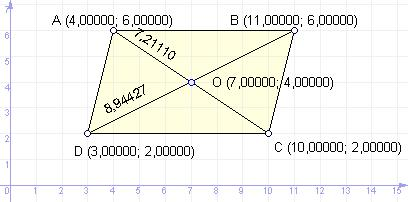Problems

# Diagonals

Four pointsA(x1; y1), B(x2; y2), C(x3; y3), D(x4; y4)are the vertices of parallelogram. Determine the length of the diagonals and find the coordinates of their intersection.Input

In 4-lines through the gap defined x and y coordinates of successive peaks parallelogram, respectively points A, B, C and D. All numbers in absolute value does not exceed 100.

Output

The first line print after a gap of x and y coordinates of the point O, in the second - print the length of the diagonal ACand through a gap - BD. The results lead to three decimal.

Time limit 1 second
Memory limit 64 MiB
Input example #1
4 6
11 6
10 2
3 2

Output example #1
7.000 4.000
7.211 8.944

Input example #10
-100 100
100 100
100 -100
-100 -100

Output example #10
0.000 0.000
282.843 282.843